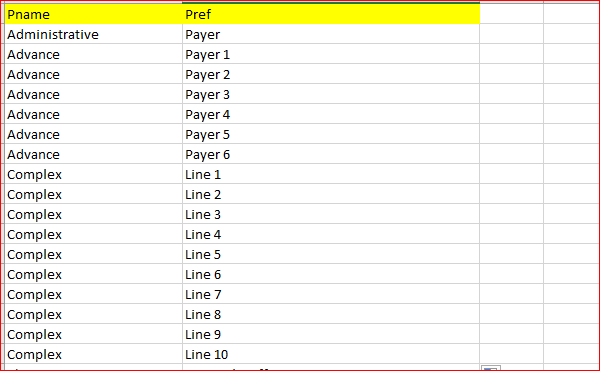# Read data from excel sheet into 2D Arraylist

Hi there,

I need help in getting the data into 2D array from excel sheet. I am aware on how to get the data from excelsheet into 1D array. Could you please help on the same?

All I have is the below excel sheetBelow code works fine to fetch single column data but I would like to fetch both mentioned columns data into a 2D Array . Could you please help?

Object excelD= ExcelFactory.getExcelDataWithDefaultSheet(‘D:\Users\Test.xlsx’,
sheet1, true)
for (int i = 1; i <= tot; i++) {
if (excelData.getValue(‘Pname’, i) == ‘’) {
break
}

``````ExlPgm.add(excelD.getValue('Pname', i).trim())
``````

}

Is this what you want?

``````for (int i = 1; i <= tot; i++) {
...
excelD.getValue('Pref',  i).trim()  ])
}
``````

@kazurayam
This code works for 1D array. This is able to get both column data into one array . I need the data in the table format into below defined array(2D)
ArrayList[][] ExlPgm= new ArrayList[numRows][];

This line look very strange to me. I have never seen `ArrayList[][]` in any Java/Groovy tutorial documents.

I would rather write to express an 2-dimensional List:

``````List<List<Object>> exlPgm = new ArrayList<ArrayList<Object>>()
``````

@kazurayam
Thanks for the correction. But still am stuck at the same question

I do not see what is your question.

As mentioned above
“This code works for 1D array. This is able to get both column data into one array . I need the data in the table format into below defined array(2D)”

List<List> exlPgm = new ArrayList<ArrayList>()

So if I have the above 2D array defined and want to fetch the data from the excel sheet mentioned above then what is the way to do it ?

Make a Test Case and copy&page the following, and run it.

``````List<List<Object>> exlPgm = [
["Complex", "Line 1"]
]

println "--------------- demo 1 ----------------------"
for (int i = 0; i < exlPgm.size(); i++) {
List<Object> row = exlPgm.get(i)
for (int j = 0; j < row.size(); j++) {
println "i=\${i},j=\${j} \'" + row.get(j) + "\'"
}
}

println "\n--------------- demo 2 ----------------------"
for (int i = 0; i < exlPgm.size(); i++) {
for (int j = 0; j < exlPgm[i].size(); j++) {
println "[\${i}][\${j}] \'" + exlPgm[i][j] + "\'"     // array-like syntax sugar
}
}

println "\n--------------- demo 3 ----------------------"
// list.each { groovy closure}
exlPgm.eachWithIndex { row, i ->
row.eachWithIndex { cell, j ->
println "[\${i}][\${j}] \'" + cell + "\'"
}
}

``````

You will see the following output:

``````--------------- demo 1 ----------------------
i=0,j=1 'Payer'
i=1,j=1 'Payer 1'
i=2,j=0 'Complex'
i=2,j=1 'Line 1'

--------------- demo 2 ----------------------
 'Payer'
 'Payer 1'
 'Complex'
 'Line 1'

--------------- demo 3 ----------------------